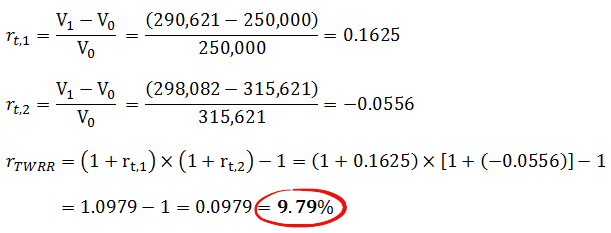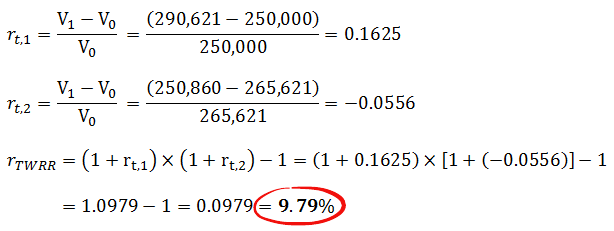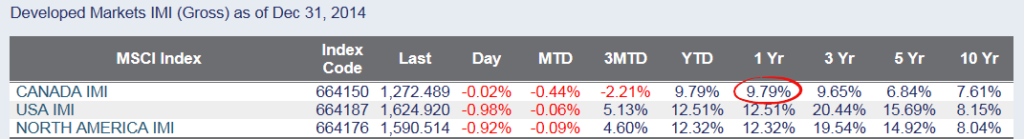The Holy Grail of portfolio performance benchmarking is the time-weighted rate of return (TWRR).  However, it requires daily portfolio valuations whenever an external cash flow (i.e. a contribution or withdrawal) occurs.  Periods in which external cash flows occur are divided into sub-periods, each with its own total return calculation.  These sub-period returns are then geometrically linked together to obtain the time-weighted rate of return over the measurement period (“geometric linking” is just a fancy way of saying “add 1 to each sub-period return, multiply the sub-period returns together, and then subtract 1 from the result”).  The daily valuation requirement makes it very difficult for the average investor to calculate their time-weighted rate of return without the help of computational software.In our initial example (please refer to my blog post on How to Calculate Your Portfolio’s Rate of Return), Investor 1 initially invested \$250,000 on December 31, 2013.  On September 15, 2014, their portfolio was worth \$290,621.  They then added \$25,000 to the portfolio, bringing the portfolio value up to \$315,621.  By the end of 2014, the portfolio had decreased to \$298,082.

Investor 1 would start by calculating their first sub-period return from December 31, 2013 to September 15, 2014 (using portfolio values before the cash flow occurred).  They would then calculate a second sub-period return from September 15, 2014 (using portfolio values after the cash flow occurred) to December 31, 2014.  After this was done, they would geometrically link the sub-period returns to obtain their time-weighted rate of return for the year.

Example:  Time-weighted rate of return for Investor 1Investor 2 initially invested \$250,000 on December 31, 2013 in the exact same portfolio as Investor 1.  On September 15, 2014, their portfolio was worth \$290,621.  They then withdrew \$25,000 from the portfolio, bringing the portfolio value down to \$265,621.  By the end of 2014, the portfolio had decreased to \$250,860.

Using the same process, Investor 2 ends up with the exact same time-weighted rate of return for the year.

Example:  Time-weighted rate of return for Investor 2Regardless of the amounts both investors contributed or withdrew from the portfolio, they ended up with the exact same return. This is precisely the result that should be expected.  The time-weighted rate of return is not affected by contributions and withdrawals into and out of the portfolio, making it the ideal choice for benchmarking portfolio managers or strategies.  If we compare their return to the returns of the MSCI Canada IMI Index over the same period (which their portfolio manager was attempting to track), we also get the same result of 9.79%.

MSCI Index Performance as of December 31, 2014##### Source:  MSCI

Before moving onto the next section, please take note of the relative difference in the sub-period returns; the first sub-period return was 16.25% before the cash flows occurred, and a relatively worse return of -5.56% after the cash flows occurred.  This difference in sub-period returns during the year is going to drive the return differences between the time-weighted rate of return and the Money-Weighted Rate of Return (MWRR).